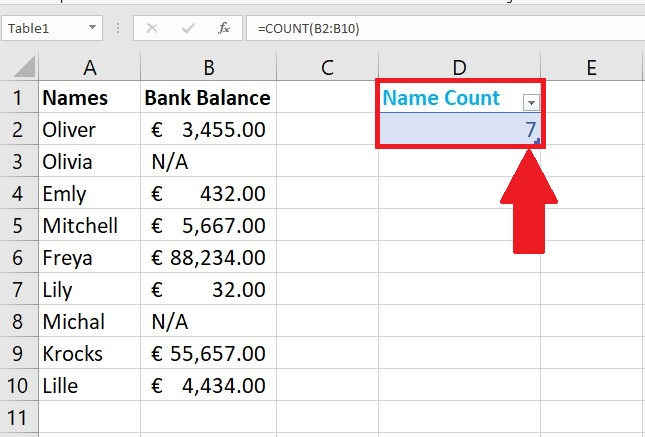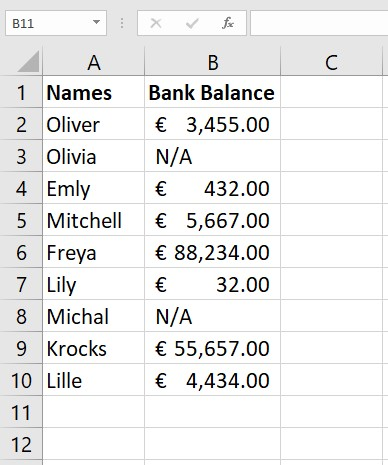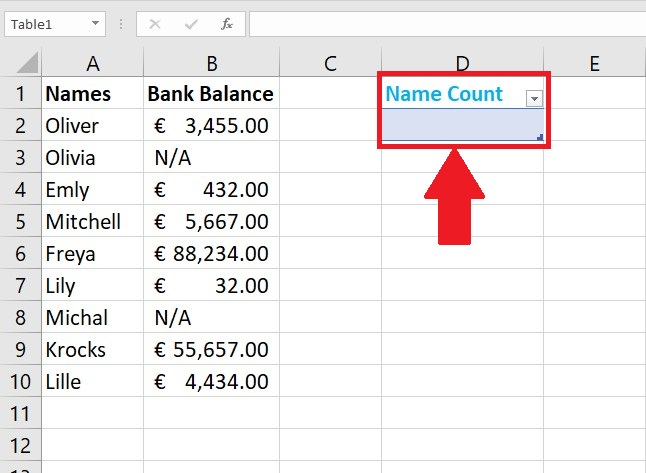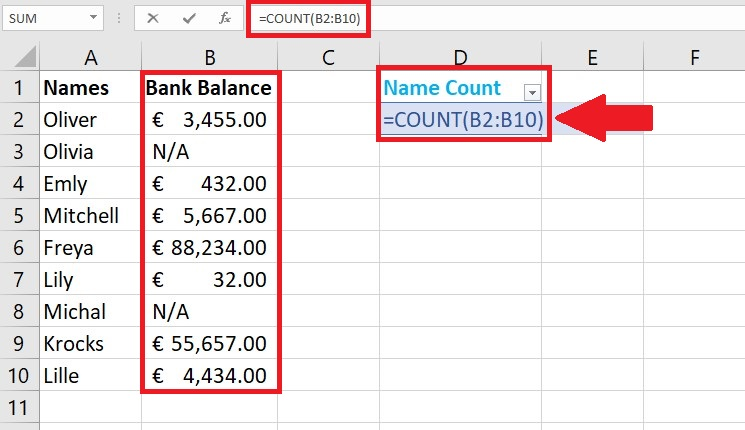# How to count names in excelIn this tutorial, we will learn how to count the names in Excel. Suppose we have a data range of names of persons and their bank balance. We want to count the names of only those people having a bank balance. Therefore, we’ll use the COUNT function of Excel for this purpose. Let’s look into the Count formula syntax and parameters first =Count(value1 : value2) – value 1 means the first data cell from where count will start. – value 2 carries the last data cell. The function counts those cells having some numeric values. It does not consider the blank cells or the cells having no value. Let’s take an example data set and implement the formula on this data set shown above;Microsoft Excel is a software that can also help us in mathematical calculations along with data analysis. There are many amazing features of Microsoft Excel which helps a lot in day to day routine. For example, COUNT is an in-built function in Excel, which will count the number of cells that contain numeric data.

### Step 1 – Create header for Count– Create a name count header.

– We will count in it, above is the example.

### Step 2 – Apply Formula– The formula that we will use to count the names in this examples is shown below;

=Count(B2:B10)

Excel will count the total number of data cells having a numeric value, picture example is shown above.

### Step 3 – Calculate the count– As soon as you press the enter key, the result is displayed as 7.

– It calculated only those NAMES having numeric value in the bank balance column.

– Names that do not contain any balance are not counted.

Hence, 7 names have been counted  that have a bank balance. The count function does not calculate the names directly. So, we calculated names by indirect method, with the support of bank balance column.